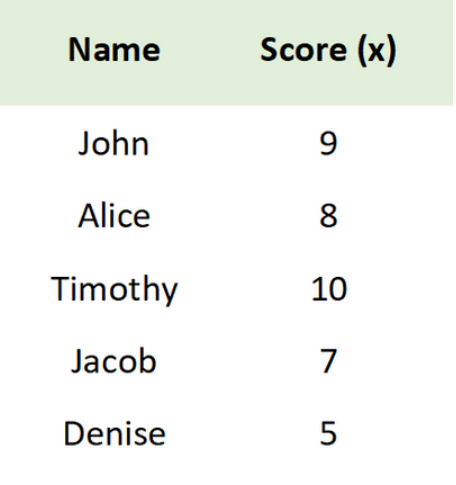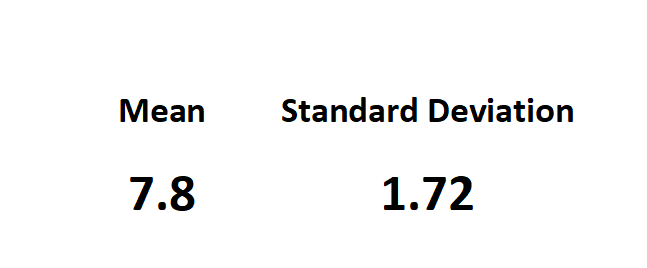top of page

# The Bell-CurveWith a dataset's average and standard deviation, we can find the probability of getting a data point of any particular value.

We can do this using the rules for normal probability, represented graphically by a bell curve.

## Normal Distribution

A "normal distribution" refers to a data set with values that occur in a bell-shaped pattern, increasing in value and frequency toward a mean, and then decreasing in frequency toward higher values.

## Skewness

A distribution that is not normal is is data set is skewed. A skewed data set has more values are concentrated either higher (right) or lower (left) than the mean. The values in a normal data set are distributed symmetrically on both sides of the mean.Income is an example of a positively (right) skewed data set. There are more people in lower income than higher income groups:Life expectancy is an example of a negatively (left) skewed data set. There are more people expected to live until higher ages than lower ages:You can test for skewness to see if your dataset is normal or skewed.

## Kurtosis

A non-normal distribution can also be skewed up "peaked" or down "flattened", meaning more values are located towards the tails (ends) or around mean (middle) of the curve. This measurement is called Kurtosis.You can test for kurtosis to see if your distribution is platykurtic or leptokurtic.

## Example

The bell shape is reflected in the outline of the graphs of a frequency distribution, such as a histogram. Here is a histogram of the test scores for a class of students in our previous example:Our data set is approximately normal (slightly skewed left and slightly platykurtic, but testing shows it is not significant).

## Using the Bell Curve to Find Probability

The bell curve of a data set with a normal (or approximately normal) distribution can be standardized so that the mean is equal to zero and each standard deviation is equal to one.The test scores are converted to z-scores - which are their number of standard deviations away from the mean.

The area under the curve is equal to 1 or 100%.

An important rules in statistics (called the Empirical Rule) states the following for all "normal" populations:

68% of the data falls within 1 standard deviation of the mean.

95% of the data falls within 2 standard deviations of the mean.

99.7% of the data falls within 3 standard deviations of the mean.Remember our example population of student test scores:Using our mean and standard deviation, we can calculate the following:68% of the scores fall between 6.08 to 8.92 points.

95% of the scores fall between 4.36 to 10.64 points.

99.7% of the scores fall within 2.64 to 12.36 points.

This method of standardizing scores (calculating how many standard deviations each score is away from the mean), allows us to find the probability of scoring particular values.

For example, we now know that there is a 95% of scoring between 4.36 to 10.64 points. Conversely, there is a 5% chance of that student a student received a score outside of that range (less than 4.36 or higher than 10.64).

Next, let's learn more about the Z-Distribution, and how to use the Z-table (or technology) to find the probability of any value in a dataset!

Contact us for practice materials regarding standard deviation, the bell curve, the empirical rule, normal probability, and more.